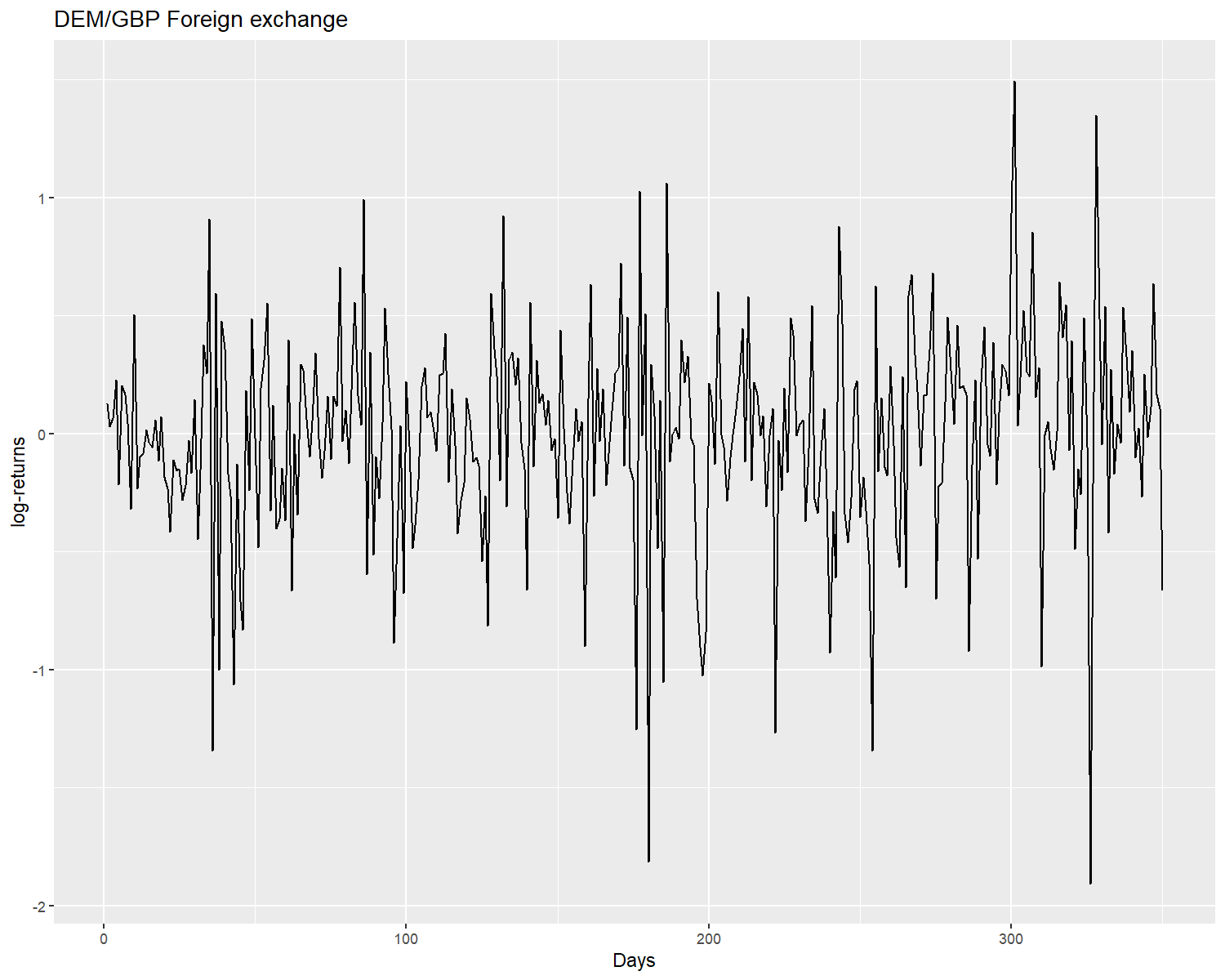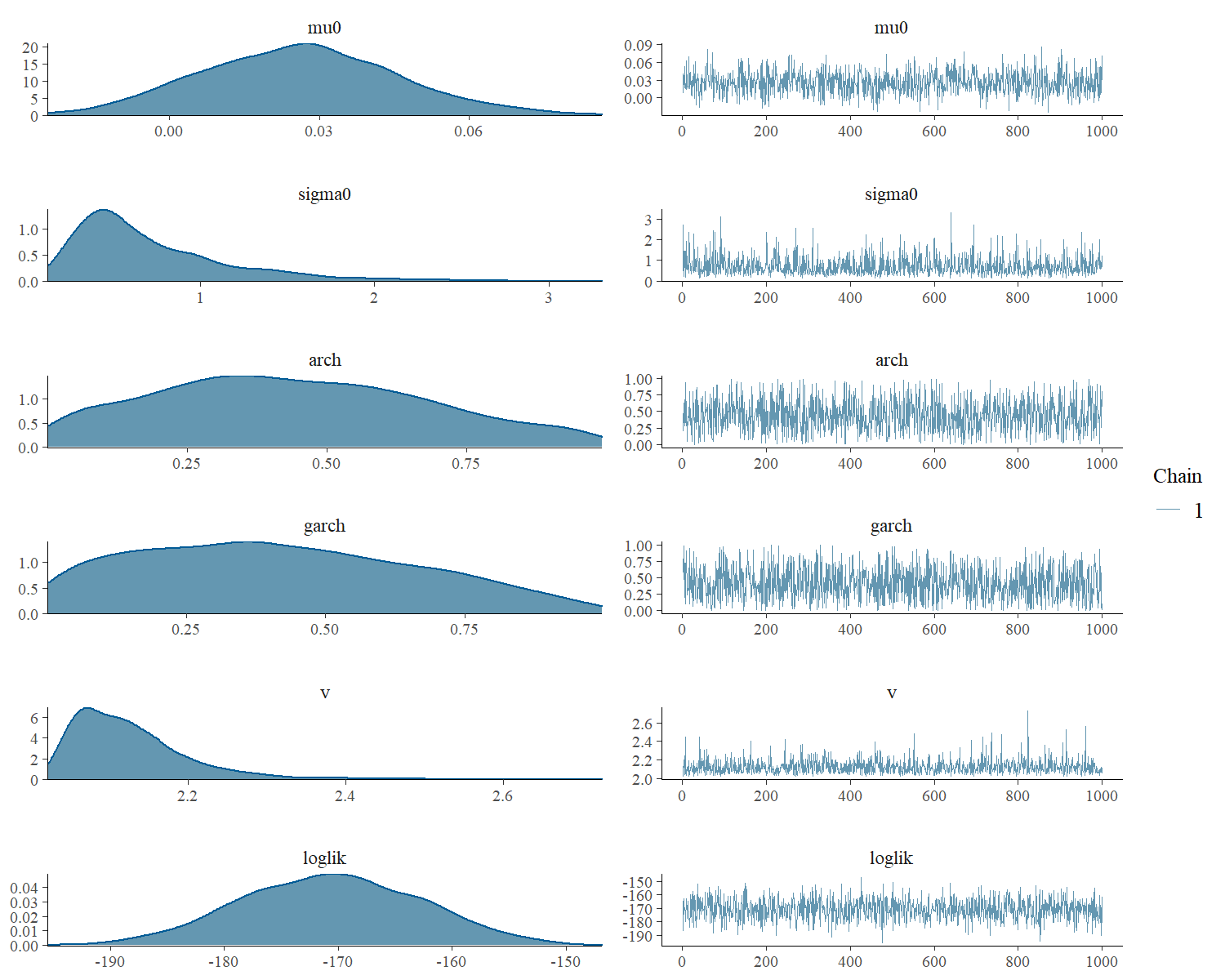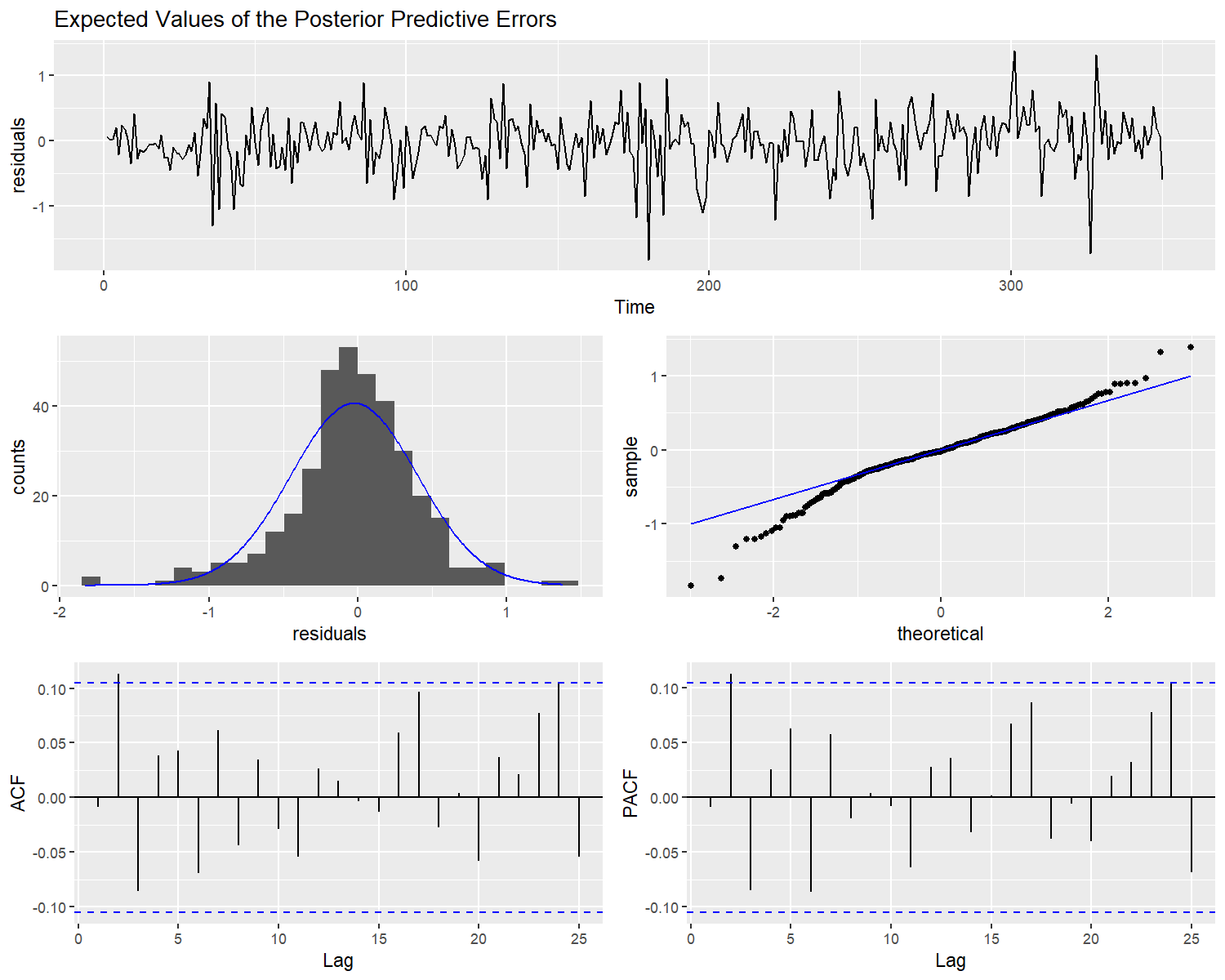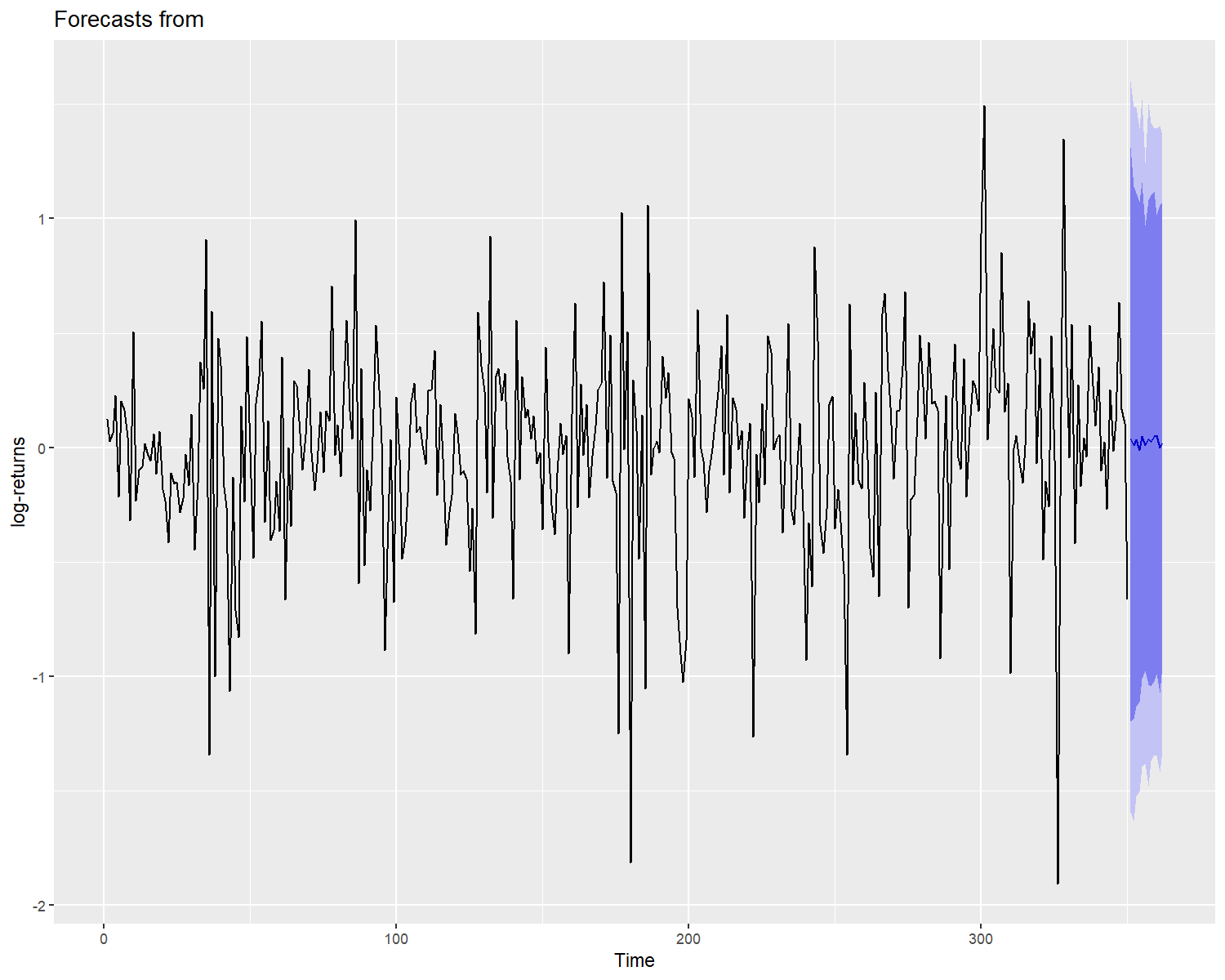Estimating GARCH Models

2021-06-17

library(bayesforecast)
library(ggplot2)

Introduction

The foreign exchange consists of converting one currency into another at a specific rate known as the foreign exchange rate. The conversion rates are constantly changing as they are driven by the market forces of supply and demand. In this vignette, we will model the volatility of the series of daily observations of the foreign exchange between Germany and the United Kingdom proposed by Ardia y Hoogerheide (2010) using the stan_garch function of the bayesforecast package. The records correspond from January 3, 1984, to December 31, 1991. However, for this work, we will use the first 350 observations.

The steps for estimating the model are:

1. Plot the data and identify any unusual observations.

2. Create de GARCH Model through the stan_garch function of the bayesforecast package.

3. Plot and observe the residuals of the model. If the residuals look like white noise, we proceed to make the prediction. Otherwise, we will choose another model. Plot the data and identify any unusual observations.

Plotting the data:

autoplot(demgbp,main ="DEM/GBP Foreign exchange",ylab ="log-returns",x = "Days" )The model proposed by Ardia y Hoogerheide (2010) in their bayesGARCH package is a GARCH(1,1) with t-student innovations of the form:

$y_t = \mu + \left(\dfrac{v-2}{v}\lambda_t \sigma_t \right)^{1/2} \epsilon_t,\\ \sigma^2_t = \sigma_0 + \alpha_1\epsilon_{t-1}^2 + \beta_1\sigma^2_{t-1}\\ \epsilon_t \sim N(0,1)\\ \lambda_t \sim IG(v/2,v/2)$

where $$\sigma_0 > 0$$,$$\alpha_1,\beta_1$$ and $$v > 2$$, moreover $$N(0,1)$$ denotes the standard normal distribution; $$IG$$ denotes the inverted gamma distribution. The restriction on the degrees of freedom parameter $$v$$ ensures the conditional variance to be finite and the restrictions on the GARCH parameters $$\sigma_0, \alpha_1$$ and $$\beta$$ guarantee its positivity.

This model can be run in bayesforecast like:

sf1 = stan_garch(ts = demgbp,order = c(1,1,0),genT = TRUE,chains = 1)
summary(sf1)
#>             mean     se        5%       95%       ess   Rhat
#> mu0       0.0260 0.0006   -0.0055    0.0588  906.8138 0.9993
#> sigma0    0.7191 0.0146    0.2305    1.6118 1043.2061 0.9991
#> arch      0.4406 0.0077    0.0542    0.8816 1045.7121 0.9990
#> garch     0.4135 0.0079    0.0376    0.8490  871.2043 1.0020
#> v         2.1271 0.0024    2.0433    2.2649 1049.2314 1.0015
#> loglik -170.6542 0.2482 -183.5434 -157.6999 1024.2370 1.0026

In the model proposed by Ardia y Hoogerheide (2010) they use truncated normal priors on the GARCH parameters $$\beta$$ and $$\alpha=(\sigma_0,\alpha_1)'$$

$p(\alpha)\propto\phi_{N_2}(\alpha|\mu_\alpha,\Sigma_\alpha) 1 \{\alpha\in R_+^2\}\\ p(\beta)\propto\phi_{N_1}(\beta|\mu_\beta,\Sigma_\beta) 1 \{\beta\in R_+\}\\$ where $$\mu_\bullet$$ and $$\Sigma_\bullet$$ are the hyperparameters, $$1\{\bullet\}$$ is the indicator function and $$\phi_{N_d}$$ is the d-dimensional normal density.

The prior distribution of vector $$\lambda=(\lambda_1,...\lambda_T)'$$ conditional on $$v$$ is found by noting that the components $$\lambda_t$$ are independent and identically distributed from the inverted gamma, which yields

$p(\lambda|v)=\left(\frac{v}{2} \right)^{\frac{Tv}{2}}\left[ \Gamma\left(\frac{v}{2}\right)\right]^{-T}\left(\prod_{t=1}^T\lambda_t\right)^{-\frac{v}{2}-1}exp\left[-\frac{1}{2}\sum_{t=1}^T\frac{v}{\lambda_t}\right]$ furthermore the prior distribution on the degrees of freedom parameters is a translated exponencial with parameters $$\lambda^*>0$$ y $$\delta\geq2$$

$p(v)=\lambda^*exp[-\lambda^*(v-\delta)]1\{v>\delta\}$ The priors used by Ardia y Hoogerheide (2010) are slightly similar to the prior by default in bayesforecast, these were defined by default following the recommendations proposed by Stan.

prior_summary(sf1)
#>
#> y ~ garch(1,1,0)
#> Generalized t-student model
#> 350 observations and 1 dimension
#>
#> Priors:
#>  Intercept:
#>  "mu0 [  ] ~ normal ( mu =  0 ,sd =  1 )"
#>
#>  Scale Parameter:
#>  "sigma0 [  ] ~ student ( mu =  0 ,sd =  1 ,df =  7 )"
#>
#>      [,1]
#> [1,] "arch [ 1 ] ~ normal ( mu =  0 ,sd =  0.5 )"
#>      [,1]
#> [1,] "garch [ 1 ] ~ normal ( mu =  0 ,sd =  0.5 )"
#>
#>  Generalized t-student
#>
#>  lambda ~ G(v/2,v/2)
#>  "df [  ] ~ gamma ( shape =  2 ,rate =  0.1 )"

Based on these previously defined parameters, the obtained posteriors are:

mcmc_plot(sf1)It is observed that the posteriors converge. Additionally, when following the model’s fit, it does not entirely fit the data because the model does not capture the dependency structure in the location parameter. Then we proceed to review the model residuals. The check_residuals function estimates the posterior mean of the residuals and plots them. Note that the plot is not sufficient to corroborate the normality and stationarity assumptions, but they are an initial indicator of the adjustment.

check_residuals(sf1)The residual series (Upper part) seems to be stationary. However, the histogram and quantile graph (middle part) show that the model has heavy tails due to the series’s high volatility.

Forecast

Based on the previous results, we finally predict the model for the next few days:

autoplot(object = forecast(sf1,h = 12),ylab="log-returns")